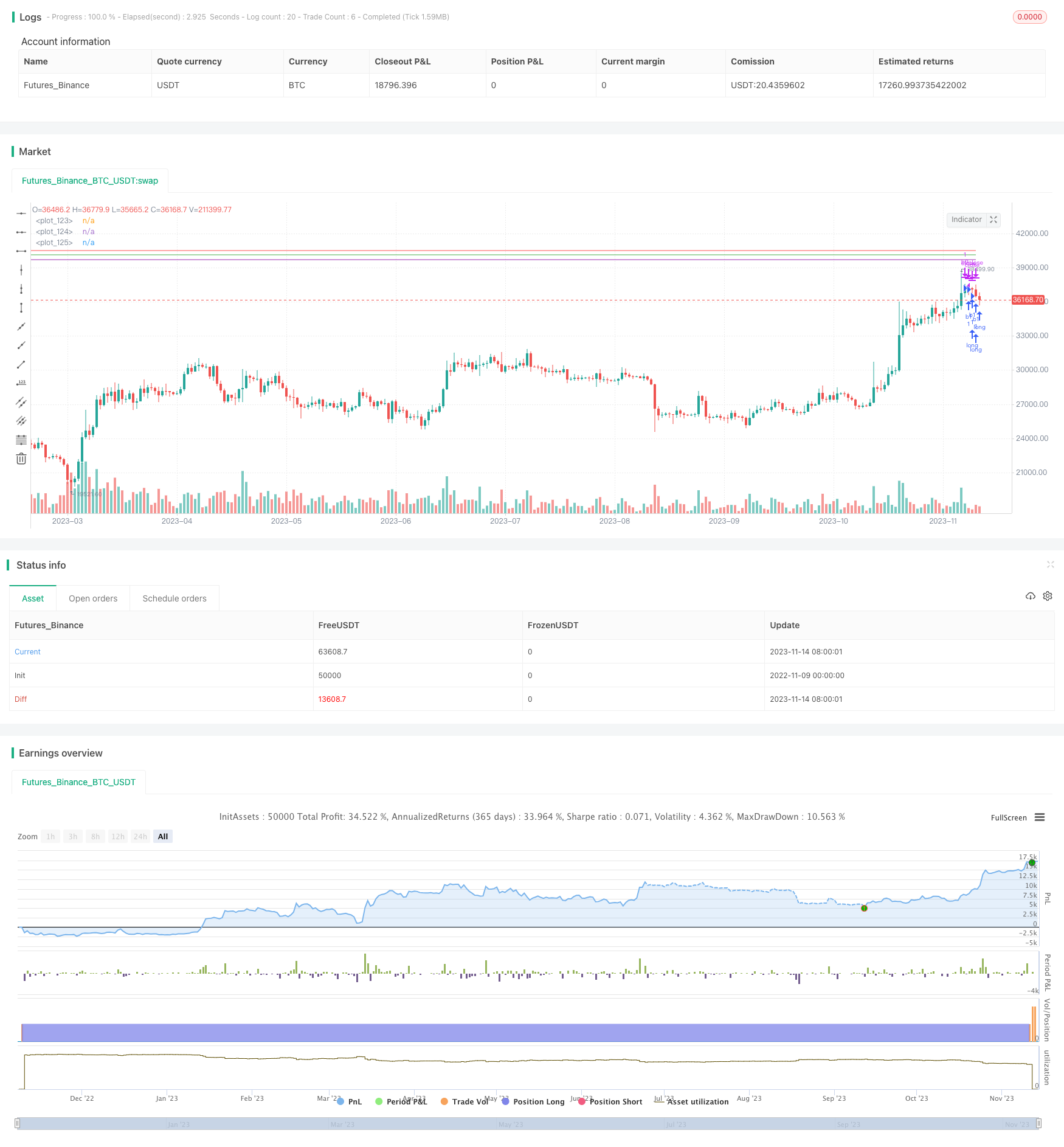Author: ChaoZhang, Date: 2023-11-16 17:09:45
Tags:## Overview

This strategy adopts fixed grid trading approach by setting the starting price and percentage between each grid layer. Then it calculates 10 fixed buy and sell prices based on the percentage to implement low-buy-high-sell grid trading strategy.

## Strategy Logic

The strategy first sets the starting price sprice and grid distance percentage gridpercent. Then it calculates 10 layers of buy and sell prices based on the starting price and percentage.

b1=sprice-(sprice*p1)

b2=sprice-(sprice*p2)

b3=sprice-(sprice*p3)

Where p1~p10 are percentages calculated layer by layer based on gridpercent.

Sell price formula:

s1=b1+(sprice*p1)

s2=b2+(sprice*p1)

s3=b3+(sprice*p1)

The buy condition triggers when close price is lower than the buy price:

if (close<b1)

strategy.entry(“b1”, strategy.long, when=(close<b1))

Similarly, sell condition triggers when close price is higher than sell price:

if (close>s1)

strategy.exit("b1", when=(close>s1))

The fixed grid strategy has the following advantages:

2. Setting proper grid distance effectively controls risk and avoids chasing.

3. Profitable whether market goes up or down.

4. Flexibility to adjust grid params for different market conditions.

5. Scaling up position size by adding grid layers.

6. Incorporating stop loss avoids huge loss in extreme market events.

## Risks

The strategy also has some risks:

1. Trading fees eat up profit during range-bound market.

2. Improper starting price and grid settings lead to loss.

3. Price gap may cause loss during extreme events.

4. Mechanical trading has order insertion risk.

5. Concentrated events may amplify losses.

Solutions:

1. Optimize grid params to ensure profit > fees.

2. Backtest to find optimal starting price and grid distance.

3. Add stop loss to control risks.

4. Relax order price to avoid insertion.

5. Set risk control to limit max loss.

## Enhancements

The strategy can be enhanced in the following ways:

1. Dynamically adjust grid distance based on volatility.

2. Compute price range to dynamically set starting price.

4. Optimize stop loss based on historical stop loss points.

5. Incorporate position sizing based on profit level.

6. Optimize position management to maximize capital utilization.

7. Improve execution using TWAP to reduce impact cost.

## Conclusion

The strategy implements fixed grid trading by setting buy and sell prices based on starting price and grid percentage, achieving auto low-buy-high-sell. It’s important to manage risks by optimizing parameters, dynamic adjustments and stop loss for profit locking and loss control. Incorporating advanced ML and money management techniques can further improve strategy profitability and win rate.

```/*backtest
start: 2022-11-09 00:00:00
end: 2023-11-15 00:00:00
period: 1d
basePeriod: 1h
exchanges: [{"eid":"Futures_Binance","currency":"BTC_USDT"}]
*/

// This source code is subject to the terms of the Mozilla Public License 2.0 at https://mozilla.org/MPL/2.0/

//@version=5
strategy("Grid HW", overlay = true, margin_long = 1, margin_short = 1)

// Fix 35k price as starting point and 1% as a distance

sprice=input(40500,"Starting price")
gridpercent=input(1,"Percent")

// calculate the % of the 10 layers

p1=((gridpercent*1)/100)
p2=((gridpercent*2)/100)
p3=((gridpercent*3)/100)
p4=((gridpercent*4)/100)
p5=((gridpercent*5)/100)
p6=((gridpercent*6)/100)
p7=((gridpercent*7)/100)
p8=((gridpercent*8)/100)
p9=((gridpercent*9)/100)
p10=((gridpercent*10)/100)

b1=sprice-(sprice*p1)
b2=sprice-(sprice*p2)
b3=sprice-(sprice*p3)
b4=sprice-(sprice*p4)
b5=sprice-(sprice*p5)
b6=sprice-(sprice*p6)
b7=sprice-(sprice*p7)
b8=sprice-(sprice*p8)
b9=sprice-(sprice*p9)
b10=sprice-(sprice*p10)

//set sell prices

s1=b1+(sprice*p1)
s2=b2+(sprice*p1)
s3=b3+(sprice*p1)
s4=b4+(sprice*p1)
s5=b5+(sprice*p1)
s6=b6+(sprice*p1)
s7=b7+(sprice*p1)
s8=b8+(sprice*p1)
s9=b9+(sprice*p1)
s10=b10+(sprice*p1)

//Long conditions

lc1=close<b1
lc2=close<b2
lc3=close<b3
lc4=close<b4
lc5=close<b5
lc6=close<b6
lc7=close<b7
lc8=close<b8
lc9=close<b9
lc10=close<b10

//exit conditions
ec1=close>s1
ec2=close>s2
ec3=close>s3
ec4=close>s4
ec5=close>s5
ec6=close>s6
ec7=close>s7
ec8=close>s8
ec9=close>s9
ec10=close>s10

//long orders
if (lc1)
strategy.entry("b1", strategy.long, when=(lc1))

if (lc2)
strategy.entry("b2", strategy.long, when=(lc2))

if (lc3)
strategy.entry("b3", strategy.long, when=(lc3))
if (lc4)
strategy.entry("b4", strategy.long, when=(lc4))
if (lc5)
strategy.entry("b5", strategy.long, when=(lc5))
if (lc6)
strategy.entry("b6", strategy.long, when=(lc6))
if (lc7)
strategy.entry("b7", strategy.long, when=(lc7))
if (lc8)
strategy.entry("b8", strategy.long, when=(lc8))
if (lc9)
strategy.entry("b9", strategy.long, when=(lc9))
if (lc10)
strategy.entry("b10", strategy.long, when=(lc10))

//exit orders
if (ec1)
strategy.exit("b1", when=(ec1), limit=1)
if (ec2)
strategy.exit("b2", when=(ec2), limit=1)
if (ec3)
strategy.exit("b3", when=(ec3), limit=1)
if (ec4)
strategy.exit("b4", when=(ec4), limit=1)
if (ec5)
strategy.exit("b5", when=(ec5), limit=1)
if (ec6)
strategy.exit("b6", when=(ec6), limit=1)
if (ec7)
strategy.exit("b7", when=(ec7), limit=1)
if (ec8)
strategy.exit("b8", when=(ec8), limit=1)
if (ec9)
strategy.exit("b9", when=(ec9), limit=1)
if (ec10)
strategy.exit("b10", when=(ec10), limit=1)

plot(b1,color=color.green)
plot(s1, color=color.red)
plot(b2, color=color.purple)
```

More